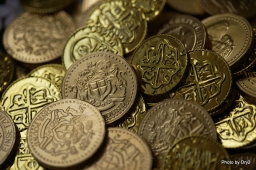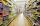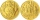# Dividing money

Vilem, Cenek, and Edita divided the money they earned by spreading the leaflet. Vilem got 240 CZK more than Cenek and twice more than Edita. Edita got 400kc less than Vilem.

Result

C =  560
V =  800
x =  400
s =  1760 Kc

#### Solution:

V=240+C
V = 2x
x = V-400

C-V = -240
V-2x = 0
V-x = 400

C = 560
V = 800
x = 400

Calculated by our linear equations calculator.
$s = V+C+x = 800+560+400 = 1760 = 1760 \ \text { Kc }$Our examples were largely sent or created by pupils and students themselves. Therefore, we would be pleased if you could send us any errors you found, spelling mistakes, or rephasing the example. Thank you!

Leave us a comment of this math problem and its solution (i.e. if it is still somewhat unclear...):Be the first to comment!#### Following knowledge from mathematics are needed to solve this word math problem:

Do you have a linear equation or system of equations and looking for its solution? Or do you have quadratic equation?

## Next similar math problems:

1. PurchaseThree buyers pay € 468. The first paid 3-times more than second, third half over second. How many euros paid each of them?
2. Far countryIn a country far away, the value of 3 pesos is 12 centavos more than the value of 1 peso. How many centavos is 1 peso worth?
3. Forest nurseryIn the forest nursery after winter, they found that 1/10 stems died out of them. For them, they land 193 new spruces. How many spruces are in the forest nursery?
4. Find xSolve: if 2(x-1)=14, then x= (solve an equation with one unknown)
5. Eq1Solve equation: 4(a-3)=3(2a-5)
6. If-then equationIf 5x - 17 = -x + 7, then x =Added together and write as decimal number: LXVII + MLXIV
8. Simple equation 1035= 7*3*x what is x?
9. The math testThe math test contains 20 problems. For each correctly solved problem, the solver gets 3 points, for each incorrectly solved or unsolved problem, 2 points are deducted. Ondrej got 25 points. How many problems did he solve correctly?
10. BenchesThe park has 64 benches. Occupied are by 18 more than empty. How many benches are occupied and empty ?
11. PopsiclesFrancis went to buy ice lollies. If he buy 8 popsicles he missed 4 USD. When he buy 7 popsicles, got back 1 USD. How many USD was a popsicle?
12. Equation 29Solve next equation: 2 ( 2x + 3 ) = 8 ( 1 - x) -5 ( x -2 )
13. Simple equation 9Solve the following equation: -8y+5=-9y+9
14. Simple equation 8Solve the following equation: 36=-(1+7x)-6(-7-x)
15. Apples 3Julka has 5 apples more than Hugo and four apples less than Annie. Hugo has 17 apples. How many apples has Julka and how Annie?
16. Unknown number 11That number increased by three equals three times itself?
17. CagesHonza had three cages (black, silver, gold) and three animals (guinea pig, rat and puppy). There was one animal in each cage. The golden cage stood to the left of the black cage. The silver cage stood on the right of the guinea pig cage. The rat was in the